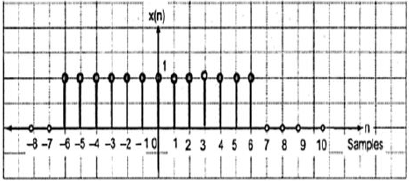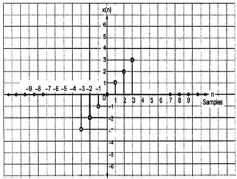# DSP - Classification of DT Signals

Just like Continuous time signals, Discrete time signals can be classified according to the conditions or operations on the signals.

## Even and Odd Signals

### Even Signal

A signal is said to be even or symmetric if it satisfies the following condition;

$$x(-n) = x(n)$$Here, we can see that x(-1) = x(1), x(-2) = x(2) and x(-n) = x(n). Thus, it is an even signal.

### Odd Signal

A signal is said to be odd if it satisfies the following condition;

$$x(-n) = -x(n)$$From the figure, we can see that x(1) = -x(-1), x(2) = -x(2) and x(n) = -x(-n). Hence, it is an odd as well as anti-symmetric signal.

## Periodic and Non-Periodic Signals

A discrete time signal is periodic if and only if, it satisfies the following condition −

$$x(n+N) = x(n)$$

Here, x(n) signal repeats itself after N period. This can be best understood by considering a cosine signal −

$$x(n) = A \cos(2\pi f_{0}n+\theta)$$ $$x(n+N) = A\cos(2\pi f_{0}(n+N)+\theta) = A\cos(2\pi f_{0}n+2\pi f_{0}N+\theta)$$ $$= A\cos(2\pi f_{0}n+2\pi f_{0}N+\theta)$$

For the signal to become periodic, following condition should be satisfied;

$$x(n+N) = x(n)$$ $$\Rightarrow A\cos(2\pi f_{0}n+2\pi f_{0}N+\theta) = A \cos(2\pi f_{0}n+\theta)$$

i.e. $2\pi f_{0}N$ is an integral multiple of $2\pi$

$$2\pi f_{0}N = 2\pi K$$ $$\Rightarrow N = \frac{K}{f_{0}}$$

Frequencies of discrete sinusoidal signals are separated by integral multiple of $2\pi$.

## Energy and Power Signals

### Energy Signal

Energy of a discrete time signal is denoted as E. Mathematically, it can be written as;

$$E = \displaystyle \sum\limits_{n=-\infty}^{+\infty}|x(n)|^2$$

If each individual values of $x(n)$ are squared and added, we get the energy signal. Here $x(n)$ is the energy signal and its energy is finite over time i.e $0< E< \infty$

### Power Signal

Average power of a discrete signal is represented as P. Mathematically, this can be written as;

$$P = \lim_{N \to \infty} \frac{1}{2N+1}\displaystyle\sum\limits_{n=-N}^{+N} |x(n)|^2$$

Here, power is finite i.e. 0<P<∞. However, there are some signals, which belong to neither energy nor power type signal.